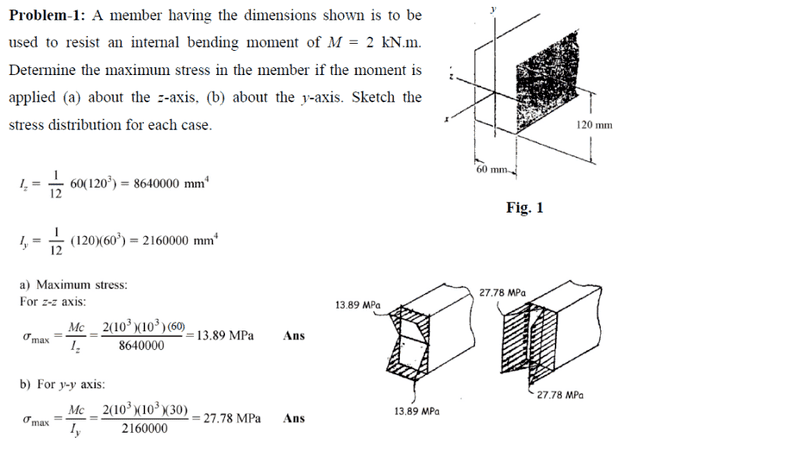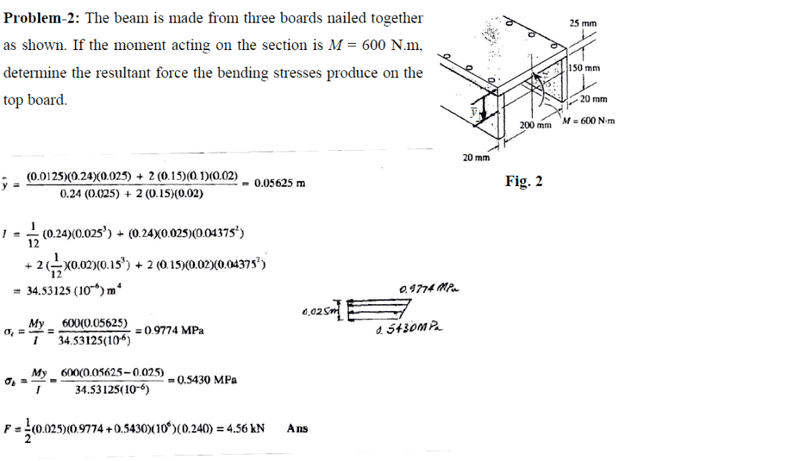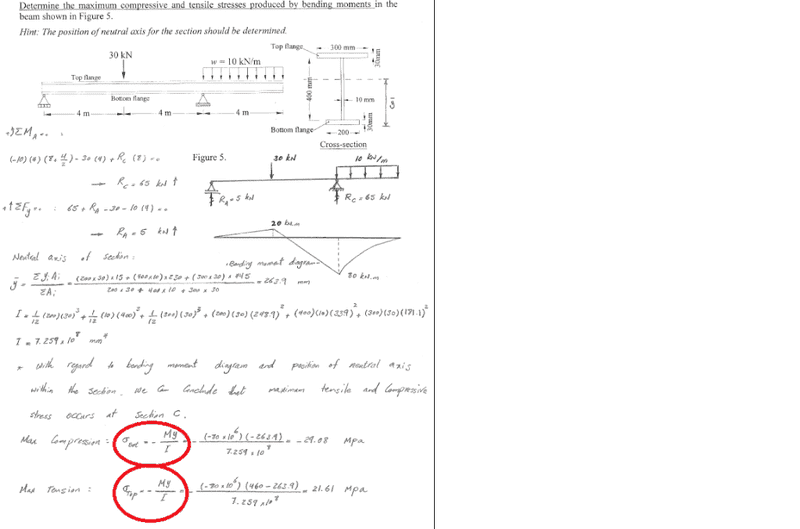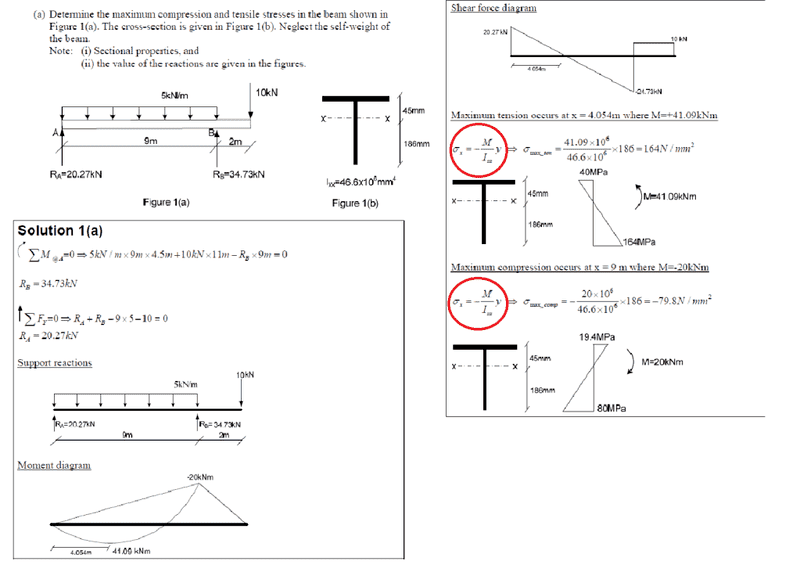# Obtaining compressive and tensile stresses in beams

hashman

## Homework Statement

In calculating stresses, how do we determine when we have a negative in front of the question and when we do not have it. I am really confused, meaning I have not understood the concept, but the examples I am looking at some have got σ= My/I whilst others have σ= -My/I.

## Homework Equations

σ= My/I

σ= -My/I

Hopefully they get uploaded properly. The first two use the first equation, whilst the the other two use the second equationThanks for the help.

LabGuy330
Just understand that:
• Compression is always Negative
• Tension is always Positive

I always just keep my numbers positive in calculation until the end and if I am solving for a compressive stress I add the negative.

Hope this helps!

hashman
Just understand that:
• Compression is always Negative
• Tension is always Positive

I always just keep my numbers positive in calculation until the end and if I am solving for a compressive stress I add the negative.

Hope this helps!

Ah right okay. So basically if they ask for max or anything always use σ=My/I , and then if it is compression, add the negative sign to the final answer. Cheers mate

LabGuy330
Yup you got it!